Year 10 Interactive Maths - Second Edition

## Elimination Method

To solve the simultaneous equations, make the coefficients of one of the variables the same value in both equations. Then either add the equations or subtract one equation from the other (whichever is appropriate) to form a new equation that only contains one variable. This is referred to as eliminating the variable.

Solve the equation thus obtained. Then substitute the value found for the variable in one of the given equations and solve it for the other variable. Write the solution as an ordered pair.

#### Example 4

Solve the following simultaneous equations by using the elimination method: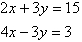##### Solution:

Label the equations as follows: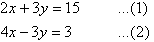Notice that 3y appears on the left-hand side of both equations.  Adding the left-hand side of (1) and (2), and then the right-hand sides, gives: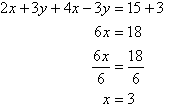###### Note: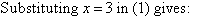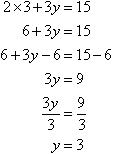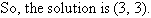#### Example 5

Solve the following simultaneous equations by using the elimination method: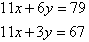##### Solution:

Label the equations as follows: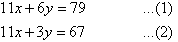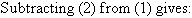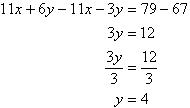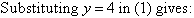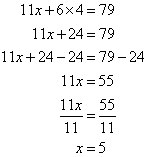So, the solution is (5, 4)

#### Example 6

Solve the following simultaneous equations by using the elimination method: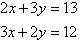##### Solution:

Label the equations as follows: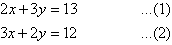Multiplying (1) by 2 and (2) by 3 gives: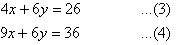Subtracting (3) from (4) gives: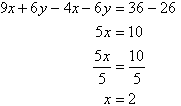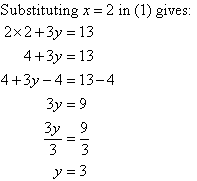So, the solution is (2, 3).

### Equations Containing Fractions

If either or both equations contain fractions, then it is desirable to remove the denominators before solving them simultaneously.

#### Example 7

Solve the following simultaneous equations by using the elimination method: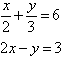##### Solution:

Label the equations as follows: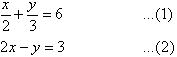To simplify equation (1), multiply both sides by 6, the lowest common denominator of 2 and 3.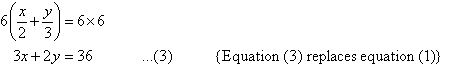The equations are now: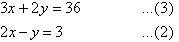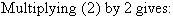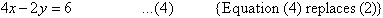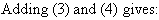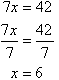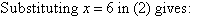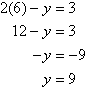So, the solution is (6, 9).